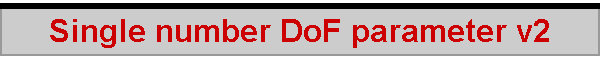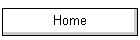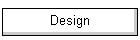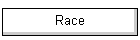This is a second version of my attempt to define a simple way of estimating depth of field in photography.  Version 1 was a little complicated and is described on the Single number DoF estimator page, with some possibly useful comments at the end on remembering the required details.  Hopefully this is a simpler and more useful approach.  Download the spreadsheet here.

We know that depth of field (DoF) depends on the focal length of the lens, distance to the subject, lens aperture, and the circle of confusion. Photo Pills provides a handy chart on its Web site and a nice calculator app for a smartphone. If you have an internet connection or have no time pressure, great. On the other hand, sat at the table in the café wondering what aperture is needed to get a reasonably in-focus shot of your two companions as the birthday cake approaches, it is clear that this doesn’t help. Surely, I thought, there could be a simple number for a given lens which, with a little mental arithmetic, would estimate the DoF with acceptable error?

Given a specific focal length, my idea is to have a simple parameter representing the depth of field at f/1 with the subject at 1 metre. From there, it should be simple to multiply up to the aperture in use and to the actual subject distance to get an estimate of the DoF.

# Near DoF Plane Only

Gazing at DoF tables quickly shows infinity cropping up pretty promptly because the far DoF plane runs off to infinity with the sniff of a short lens or a stopped down aperture, while the near DoF plane never does. So the first decision is that the simple parameter should estimate the near part of DoF only, and doubling or trebling it would then extend it to provide an overall DoF estimate (if the subject was nowhere near the hyperfocal distance).

# Linear in f, quadratic in distance

It turns out that near DoF varies as the aperture, but also as the square of the subject distance. A single parameter for near DoF would need to be multiplied by the aperture in use and then multiplied twice by the actual subject distance to get an estimate of near DoF.

This is the theoretically calculated table of near DoF for a 25 mm MFT lens, that is, the DoF in front of the subject, for apertures from f/2 to f/22 and subject distances from 0.25 m to 12 m.  For example, near DoF at f/2.8 with the subject at 2 m is 237 mm: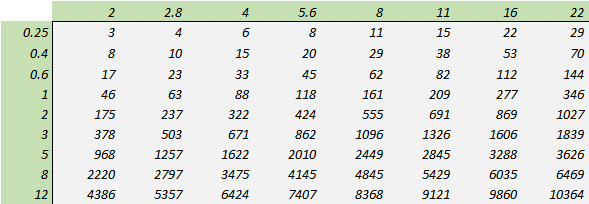This is the table of normalised parameters, where near DoF is expressed as per aperture f and per subject distance squared, that is, DoF when f=1 and SD=1: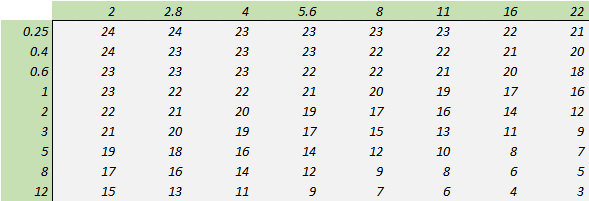The values range from 3 to 24, which does not suggest a constant value for the parameter. Restricting the table to use cases of particular interest, however, gives a much better impression. This is a table of normalised parameters oriented towards close-up photos with significant bokeh, apertures from f/2 to f/4 and subjects placed between 0.25 m and 3 m.  The values range from 24 to a much more reasonable 19: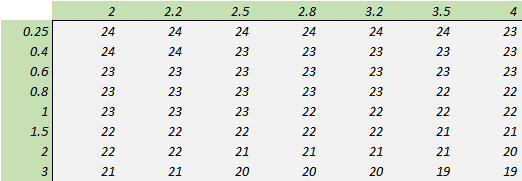The average of these values, 22.2, seems quite promising as a single value estimator. The important question is, just how accurate is it?

# Accuracy

The first step is to define how to measure and express the error in any estimate of near DoF. “Relative error” is a measure of accuracy relative relative to the size of what is being estimated or measured. For example, the actual DoF is 93 and an estimate suggests 80, the error is -13 and thus the relative error is -14%. If the actual DoF is 561 and the estimate suggests 640, the error is +79 but the relative error is still a similar magnitude at +14%.

The next step is to decide on relative to what. For example, we might consider an error of 53 mm in a DoF of 93 mm to be less important if the estimate was 146 mm, but more important if the estimate was 40 mm. In engineering terms, this is the same magnitude of error, +57% or -57%, relative to the true value, but maybe not in subjective terms.  The error might be better related to its estimate rather than to the 'true' value, in which case the error would be +36% when the estimate was larger than the true value, but -133% when less.  This approach to relative error might be called perceptual and is the one used in this analysis.

The question of accuracy can be broken down into three related but distinct questions. One is a question of the maximum error that might be seen in a set of estimates, and it might be important to ensure that this maximum is as small as possible (from game theory, this is known as the MiniMax principle, to minimise the maximum error). Two is a question of the balance of the errors, where it might be important that the overestimates should balance the underestimates. And three is a question of expressing just how large the error magnitudes are "on average".

## Maximum Error

The maximum error in a set of estimates is easily determined by finding the largest value. Computationally, this requires ignoring the sign of the error, since an error of -30% is arithmetically smaller than an error of +3%.

## Balance of Errors – 'ordinary' average

Here, the signs of the errors are taken into account in calculating the ordinary average of the errors. If the average error is close to zero, the set of errors are balanced -- the magnitudes of the under-estimates are as large as those of the over-estimates. If the average is negative, then underestimates predominate, and overestimates predominate with a positive average.

## Average Error – RMS

In calculating the 'ordinary' average of the errors, very large overestimates could be balanced by very large underestimates, leading to a very small average error masking errors of very large magnitude. A common (engineering) approach to measuring 'true' average error is RMS (root mean square). Here, the errors are first squared, their average calculated, and then the square root taken. This gives a measure of the general absolute size of the errors while giving proportionately greater weight to larger errors and allowing some statistical inferences about the probabilities of large and small errors.

# Single Parameter in use

In use, a single value parameter for near Dof of 22.8 is not much help when a quick calculation is needed while sat in the café. It needs to be nicer, rounder, number for mental arithmetic, and a value of 20 suggests itself. This is the table of relative errors in the estimated near DoF using a value of 20 for the NDp parameter: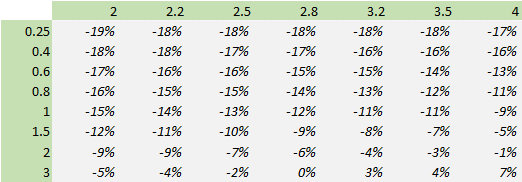For the given use cases and rounded parameter, this is the summary of the accuracy: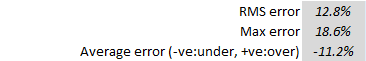Broadly speaking, these are very good results. The next nicely rounded number for NDp would be 25, and the accuracy summary shows that the balance of errors changes from 11% underestimates to 13% overestimates, with a slight increase in RMS error to 14.5% and a larger increase in the maximum error to 34%.

The key driver of accuracy in practice, however, is not the rounded NDp parameter, but my ability to estimate the subject distance of my two companions. If I guessed 1 m instead of 1.5 m with the lens at f/2, I would estimate near DoF as 40 mm instead of 90 mm, an uncertainty much larger than the nominal errors of 6 mm or 11 mm due to a rounded NDp parameter for those use cases.

# Example of macro use cases

Near DoF using the Olympus 60/2.8 Macro for close-ups between 0.25 m and 3 m and apertures between f/2.8 and f/5.6 is calculated from theory as: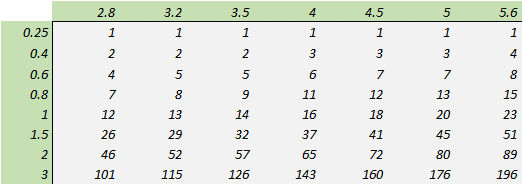Estimating near DoF using NDp = 4 as suggested by the spreadsheet yields: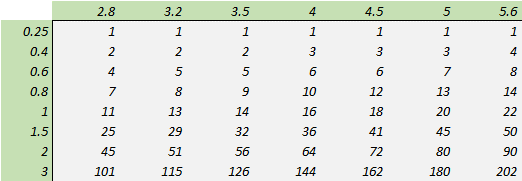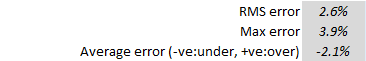Excellent agreement! All I need are careful estimates of subject distance.